### IMO Shortlist 2009 problem G6

Kvaliteta:
Avg: 3,0
Težina:
Avg: 8,0
Let the sides$AD$ and$BC$ of the quadrilateral$ABCD$ (such that$AB$ is not parallel to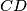$CD$) intersect at point$P$. Points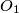$O_1$ and$O_2$ are circumcenters and points$H_1$ and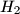$H_2$ are orthocenters of triangles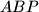$ABP$ and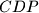$CDP$, respectively. Denote the midpoints of segments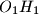$O_1H_1$ and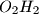$O_2H_2$ by$E_1$ and$E_2$, respectively. Prove that the perpendicular from$E_1$ on$CD$, the perpendicular from$E_2$ on$AB$ and the lines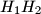$H_1H_2$ are concurrent.

Proposed by Ukraine
Izvor: Međunarodna matematička olimpijada, shortlist 2009